Courses

# RD Sharma Solutions Ex-8.4, (Part -2), Lines And Angles, Class 9, Maths Class 9 Notes | EduRev

## Class 9 : RD Sharma Solutions Ex-8.4, (Part -2), Lines And Angles, Class 9, Maths Class 9 Notes | EduRev

The document RD Sharma Solutions Ex-8.4, (Part -2), Lines And Angles, Class 9, Maths Class 9 Notes | EduRev is a part of the Class 9 Course RD Sharma Solutions for Class 9 Mathematics.
All you need of Class 9 at this link: Class 9

Q 15 : In the below fig, ∠1 = 60 and ∠2 = (2/3)rd of a right angle. Prove that l|| m.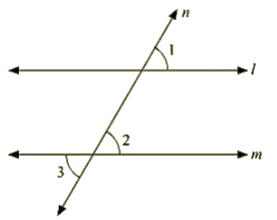Ans :     Given :

∠1 = 60 and ∠2 = (2/3)rd of a right angle

To prove : parallel Drawn to m

Proof ∠1 = 60

∠2 = (23)×90 = 60

Since ∠1 =∠1 = 60

Therefore, Parallel to m as pair of corresponding angles are equal.

16. In the below fig, if l||m||n and ∠1 = 60. Find ∠2.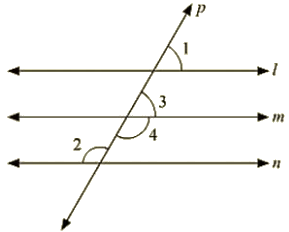Ans : Since l parallel to m and p is the transversal

Therefore, Given: l||m||n

∠1 = 60

To find ∠2

∠1=∠3 = 60∘               [Corresponding angles]

Now, ∠3and∠4 are linear pair of angles

∠3+∠4 = 180

60 + ∠4 = 180

∠4 = 180 — 60

⇒ 120

Also, m||n and P is the transversal

Therefore ∠4 = ∠2 = 120 (Alternative interior angle]

Hence 2 ∠2 = 120

Q 17 : Prove that the straight lines perpendicular to the same straight line are parallel to one another.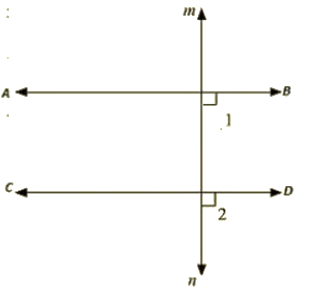Ans : Let AB and CD be drawn perpendicular to the Line MN

∠ABD = 90    [ AB is perpendicular to MN ]     —–(i)

∠CON = 90    [CD is perpendicular to MN ]      —–(ii)

Now,

∠ABD = ∠CDN = 90 [From (i) and (ii)]

Therefore,  AB||CD, Since corresponding angles are equal.

Q 18 : The opposite sides of a quadrilateral are parallel. If one angle of the quadrilateral is 601. Find the other angles.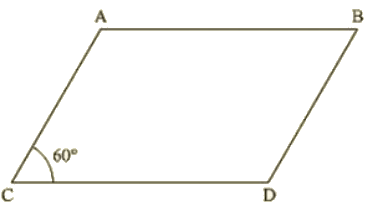Ans : Given AB || CD

Since AB || CD and AD is the transversal

Therefore, A + D = 180 (Co-interior angles are supplementary)

60 + D = 180

D = 180 – 60

D  = 120

Now. AD || BC and AB is the transversal

A + B = 180  (Co-interior angles are supplementary)

60 +B = 180

B = 180 — 60

= 120

Hence, ∠A = ∠C = 60 and ∠B =∠D = 120∘

Q 19 :  Two lines AB and CD intersect at O. If ∠AOC+∠COB+∠BOD = 270, find the measures of ∠AOC,∠COB,∠BOD,∠DOA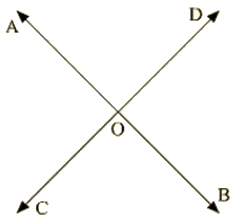Ans :

Given : ∠AOC+∠COB+∠BOD=270∘

To find : ∠AOC,∠COB,∠BOD,∠DOA

Here, ∠AOC+∠COB+∠BOD = 270  [ Complete angle]

⇒ 270 + AOD = 360

⇒ AOD = 360 — 270

⇒ AOD  =  90

Now, AOD + BOD = 180      [Linear pair]

90 + BOD = 180

⇒  BOD = 180 – 90

⇒ BOD  = 90

AOD  = BOC = 90    [Vertically opposite angles]

BOD  = AOC = 90    [Vertically opposite angles]

Q 20. In the below figure, p is a transversal to lines m and n, ∠2 = 120 and ∠5 = 60. Prove that m|| n.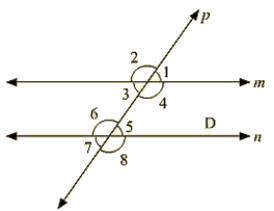Ans :

Given that

∠2 = 120 and ∠5 = 60

To prove,

∠2+∠1 = 180     [ Linear pair ]

120+∠1 = 180

∠1 = 180−120

∠1 = 60

Since ∠1 = ∠5 = 60

Therefore, m||n  [As pair of corresponding angles are equal]

Q 21 : In the below fig. transversal t intersects two lines m and n, ∠4 =110 and ∠7 = 65 Is m||n ?Ans :  Given :

∠4 = 110 and ∠7 = 65

To find : Is m||n

Here. ∠7 = ∠5 = 65            [Vertically opposite angle]

Now. ∠4+∠5 = 110+65 = 175

Therefore, m is not parallel to n as the pair of co interior angles is not supplementary.

Q 22 : Which pair of lines in the below fig. is parallel ? give reasons.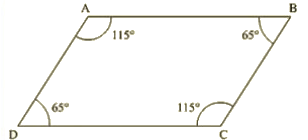Ans : ∠A+∠B = 115+65 = 180

Therefore, AB || BC [ As sum of co interior angles are supplementary]

∠B+∠C = 65+115 = 180

Therefore, AB || CD (As sum of interior angles are supplementary]

Q 23 : If I, m, n are three lines such that I|| m and n perpendicular to l, prove that n perpendicular to m.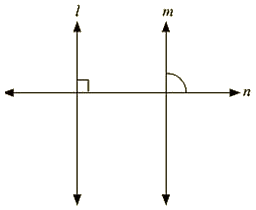Ans :

Given, l||m, n perpendicular to I

To prove: n perpendicular to m

Since l||m and n intersects

∴ ∠1 = ∠2  [Corresponding angles]

But, U = 90

⇒ ∠2 = 90

Hence n is perpendicular to m

Q 24 : In the below fig, arms BA and BC of ∠ABC are respectively parallel to arms ED and EF of∠DEF. Prove that ∠ABC = ∠DEF.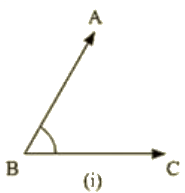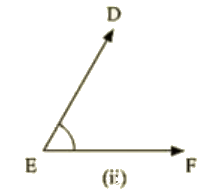Ans :

Given

AB || DE and BC || EF

To prove : ∠ABC=∠DEF

Construction: Produce BC to x such that it intersects DE at M.

Proof : Since AB || DE and BX is the transversal

ABC = DMX  [Corresponding angle]                  —–(i)

Also, BX || EF and DE Is the transversal

DMX = DEF [Corresponding angles]                —–(ii)

From (i) and (ii)

∠ABC =∠DEF

Q 25:  In the below fig, arms BA and BC of ABC are respectively parallel to arms ED and EF of DEF Prove that ∠ABC+∠DEP = 180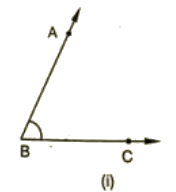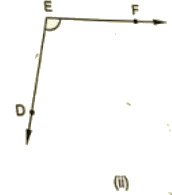Ans :

Given:

AB II DE, BC II EF

To prove: ∠ABC+∠DEF = 180

Construction: Produce BC to intersect DE at MProof :

Since AB || EM and BL is the transversal

∠ABC = ∠EML                 [Corresponding angle]  —–(i)

Also,

EF || ML and EM is the transversal

By the property of co-interior angles are supplementary

∠DEF+∠EML = 180                                      (ii)

From (i) and (ii) we have

Therefore ∠DEF+∠ABC = 180

Q 26 : With of the following statements are true (T) and which are false (F)? Give reasons.

(1) If two lines are intersected by a transversal, then corresponding angles are equal.

(ii) If two parallel lines are intersected by a transversal, then alternate interior angles are equal.

(ii) Two lines perpendicular to the same line are perpendicular to each other.

(iv) Two lines parallel to the same line are parallel to each other.

(v) If two parallel lines are intersected by a transversal, then the interior angles on the same side of the transversal are equal.

Ans :

(i) False

(ii)True

(iii) False

(iv) True

(v) False

Q 27: Fill in the blanks in each of the following to make the statement true:

(i) If two parallel lines are intersected by a transversal, then each pair of corresponding angles are ____________

(ii) If two parallel lines are intersected by a transversal, then interior angles on the same side of the transversal are _____________

(iii) Two lines perpendicular to the same line are _______ to each other

(Iv) Two lines parallel to the same line are __________ to each other.

(v) If a transversal intersects a pair of lines in such a way that a pair of alternate angles we equal. then the lines are ___________

(vi) If a transversal intersects a pair of lines in such a way that the sum of interior angles on the seine side of transversal is 180′. then the lines are _____________

Ans :

(i) Equal

(ii) Parallel

(iii) Supplementary

(iv) Parallel

(v) Parallel

(vi) Parallel

Offer running on EduRev: Apply code STAYHOME200 to get INR 200 off on our premium plan EduRev Infinity!

91 docs

,

,

,

,

,

,

,

,

,

,

,

,

,

,

,

,

,

,

,

,

,

,

,

,

,

,

,

,

,

,

,

,

,

;